Mobile QR Code1. (Department of Electronic Engineering, Hanyang University, Korea)

Time-domain, continuous-time delta-sigma modulator (CTDSM), VCO-based integrator, GRO-based quantizer, analog-to-digital converter (ADC)

## I. INTRODUCTION

A continuous-time delta-sigma modulator (CTDSM) is a popular architecture to design an analog-to-digital converter (ADC) requiring a high resolution ({\textgreater}10-bit) and a medium bandwidth ({\textless}100-MHz). In general, the operational transconductance amplifier (OTA)-based integrator and the voltage-domain quantizer are used in the CTDSM. However, it is difficult to achieve high performance of the OTA in advanced CMOS technologies, and the voltage-domain quantizer can degrade SNDR at the low supply voltage because the noise is constant but the 1-LSB voltage becomes smaller.

In order to solve these problems with voltage-domain circuits, the integrator and the quantizer in the CTDSM can be replaced with a time-domain circuit such as a VCO. The VCO-based integrator in the CTDSM has many advantages . However, when the VCO acts as a quantizer, a harmonic distortion occurs due to the nonlinear voltage-to-frequency curve of the VCO. In , the nonlinearity is alleviated by placing a high-gain integrator in front of the quantizer, but it is not easy to design the high-gain integrator. The VCO-based quantizer using the voltage-to-phase relationship can improve the linearity, but an additional dynamic element matching (DEM) logic is required to reduce the effect of the DAC mismatch . Using differential VCOs in the quantizer can achieve intrinsic clocked averaging (CLA)-based DEM, but it is more susceptible to the DAC mismatch than the data weighted averaging (DWA)-based DEM .

In this paper, the 3rd-order time-domain CTDSM using VCO-based integrators and the GRO-based quantizer is presented. Since the GRO-based quantizer can obtain 1$^{\mathrm{st}}$-order noise-shaping without increasing the order of signal transfer function (STF), the degradation of the high-frequency input is mitigated, and thus the CTDSM can operate with a high oversampling ratio (OSR). Also, the GRO-based quantizer has magnificent linearity and is less vulnerable to the DAC mismatch because of the intrinsic DWA-based DEM. Since the input of the GRO-based quantizer is a PWM signal, the voltage-to-PWM converter is required when the voltage-domain integrator is used in the CTDSM. However, this issue can be solved by the VCO-based integrator, which generates the time-domain output signal without additional circuits.

The organization of this paper is as follows. Section II compares the various VCO-based quantizers and the GRO-based quantizer. Also, section III presents the proposed 3$^{\mathrm{rd}}$-order CTDSM architecture, and section IV describes the internal circuit implementation. Finally, sections V and VI show the measurement results and the conclusions.

## II. REVIEW OF THE TIME-DOMAIN QUANTIZERS

Fig. 1 shows the architecture of the various time-domain quantizers. Fig. 1(a) shows the quantizer used in . The output phases of the VCO are sampled by the flip-flops, and the digital output proportional to the output frequency of the VCO is generated by the differentiator. The differentiator filters the quantization noise added at the sampling to the high frequency. Therefore, the 1$^{\mathrm{st}}$-order noise shaping can be obtained without the increase of STF order. Also, since the quantizer output has a DWA-based DEM pattern, the DAC mismatch has little effect on the performance of the CTDSM. However, since the range of the input voltage is large, the nonlinear gain of the VCO makes the harmonic distortion. Fig. 1(b) shows the quantizer using the relationship between the input voltage and the output phase of the VCO. This quantizer compares the output phase of the VCO to the reference phase using the XOR gate which acts as a phase detector (PD) . Therefore, the range of the input voltage is small, and the nonlinearity has little effect on the performance of the CTDSM. However, the 1$^{\mathrm{st}}$-order low pass filter is added to the STF and the DEM logic is required to prevent the SNDR degradation due to the DAC mismatch. The quantizer, as shown in Fig. 1(c), compares the output phases of the differential VCOs with an XOR gate . The advantage obtained by using the voltage-to-phase relationship is maintained and the output is expressed as a CLA-based DEM pattern. However, the CLA-based DEM is more vulnerable to the DAC mismatch than the DWA-based DEM. The quantizer in Fig. 1(d) digitizes the input pulse width modulation (PWM) signal using GRO which oscillates when the input is high and turns off when it is low. Since the differentiator is used, as like in Fig. 1(a), the digital output is proportional to the input pulse width and the 1$^{\mathrm{st}}$-order noise shaping can be added without increasing the order of the STF. Furthermore, the digital output also has the DWA-based DEM pattern and the nonlinearity issue in Fig. 1(a) can be solved since the input signal is a PWM signal, not a voltage. In the voltage-domain CTDSM, a voltage-to-PWM converter is required to use the GRO-based quantizer, but the VCO-based integrator can generate the PWM output without additional circuitry. As a result, the disadvantages that occur in the various time-domain CTDSMs can be overcome by using the VCO-based integrator and the GRO-based quantizer. The specifications of the time-domain quantizers are summarized in Table 1.

##### Fig. 1. The architecture of time-domain quantizers: (a) VCO-based quantizer using voltage-to-frequency relationship; (b) VCO-based quantizer using voltage-to-phase relationship; (c) VCO-based quantizer using differential VCOs; (d) GRO-based quantizer.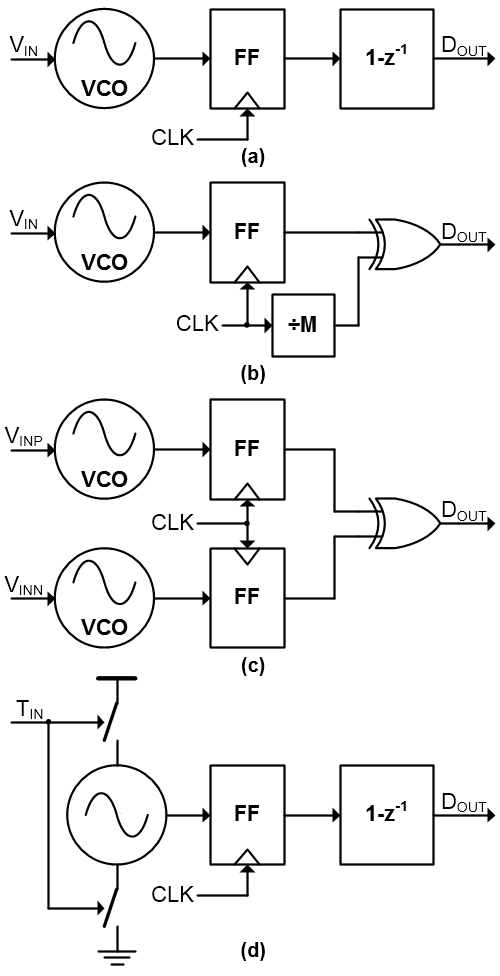##### Table 1. Comparison of the various time-domain quantizers
 STF order NTF order Linearity Intrinsic DEM Input signal Fig. 1(a) 0$^{\mathrm{th}}$ 1$^{\mathrm{st}}$ Bad DWA Voltage Fig. 1(b) 1$^{\mathrm{st}}$ 1$^{\mathrm{st}}$ Good X Voltage Fig. 1(c) 1$^{\mathrm{st}}$ 1$^{\mathrm{st}}$ Good CLA Voltage Fig. 1(d) 0$^{\mathrm{th}}$ 1$^{\mathrm{st}}$ Good DWA PWM

## III. ARCHITECTURE OF CTDSM

Fig. 2 shows the proposed 3$^{\mathrm{rd}}$-order time-domain CTDSM architecture. It consists of two VCO-based integrators, a GRO-based quantizer, DACs, and a frequency controller. First, the integrators operate based on the integral relationship between the input voltage and the output phase of the VCO. The 1$^{\mathrm{st}}$ integrator detects the phase differences between the multi-phase outputs of the differential VCOs with the phase frequency detector (PFD) and generates PWM signals proportional to the phase difference. Similar to the 1$^{\mathrm{st}}$ integrator, the 2$^{\mathrm{nd}}$ integrator generates the PWM signal with the VCO and the PFD, but only one of the VCO’s multi-phase outputs is transmitted to the PFD because only one UP and DN are needed for the GRO-based quantizer. Thus, the 2$^{\mathrm{nd}}$ integrator produces the two PWM outputs, UP and DN. These PWM signals are delivered to the GRO-based quantizer composed of the two GRO TDCs, and the GRO TDCs convert them to the digital thermometer code. The TDC has better linearity between its input and output than the voltage-to-digital converter. In addition, since the thermometer output code is generated with an intrinsic DWA mechanism, it mitigates the SNDR deterioration caused by the DAC mismatch. A DAC$_{1}$ and a DAC$_{2}$ convert the thermometer code to the analog signal for feedback. In general, a direct path to compensate for excess loop delay (ELD) is required , but it is difficult to convert thermometer code to the input PWM signal of the GRO-based quantizer directly. Therefore, the ELD is compensated by connecting the DSM output to the input of the 2$^{\mathrm{nd}}$ integrator through the differentiator. The resonator is added for optimizing the NTF. In order to generate the input PWM signal of the GRO TDC only once during a sampling period, the center frequency of the VCO$_{\mathrm{P2}}$ and the VCO$_{\mathrm{N2}}$ should be equal to the sampling frequency. For this purpose, a feedback loop to control the frequency of the VCO$_{\mathrm{P2}}$ and the VCO$_{\mathrm{N2}}$ is added.

##### Fig. 2. A proposed 3$^{\mathrm{rd}}$-order CTDSM architecture.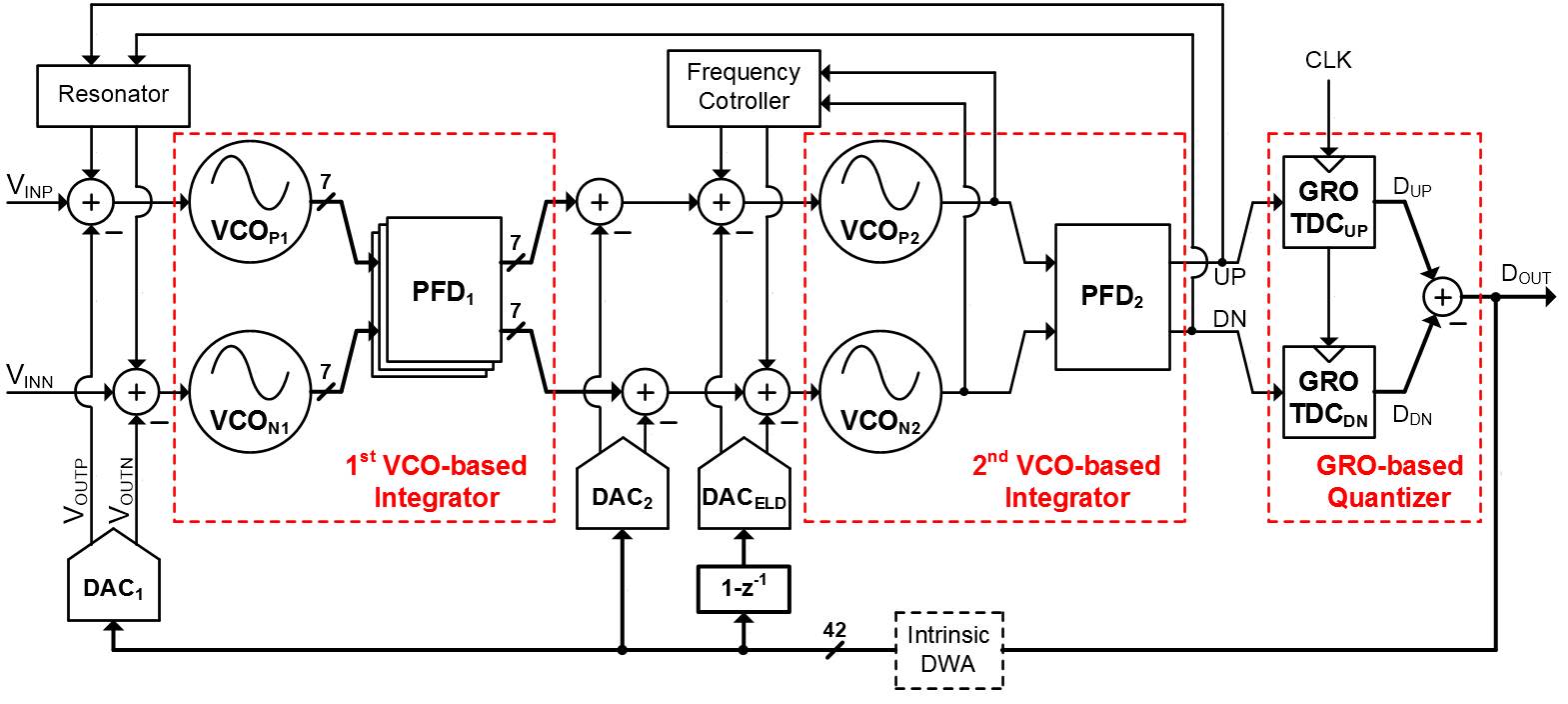## IV. CIRCUIT IMPLEMENTATIONS

### 1. VCOs in Two-stage Integrators

Fig. 3 shows the VCO schematic of the 1$^{\mathrm{st}}$-stage integrator. The digital thermometer outputs of the DSM are connected to the resistors (R$_{2}$) directly and converted to an analog voltage. This voltage is added to the input voltage at a ratio determined by R$_{1}$ and R$_{2}$. The V$_{\mathrm{X}}$ is expressed by the following equation,

##### Fig. 3. The schematic of the VCO in the 1$^{\mathrm{st}}$ stage integrator.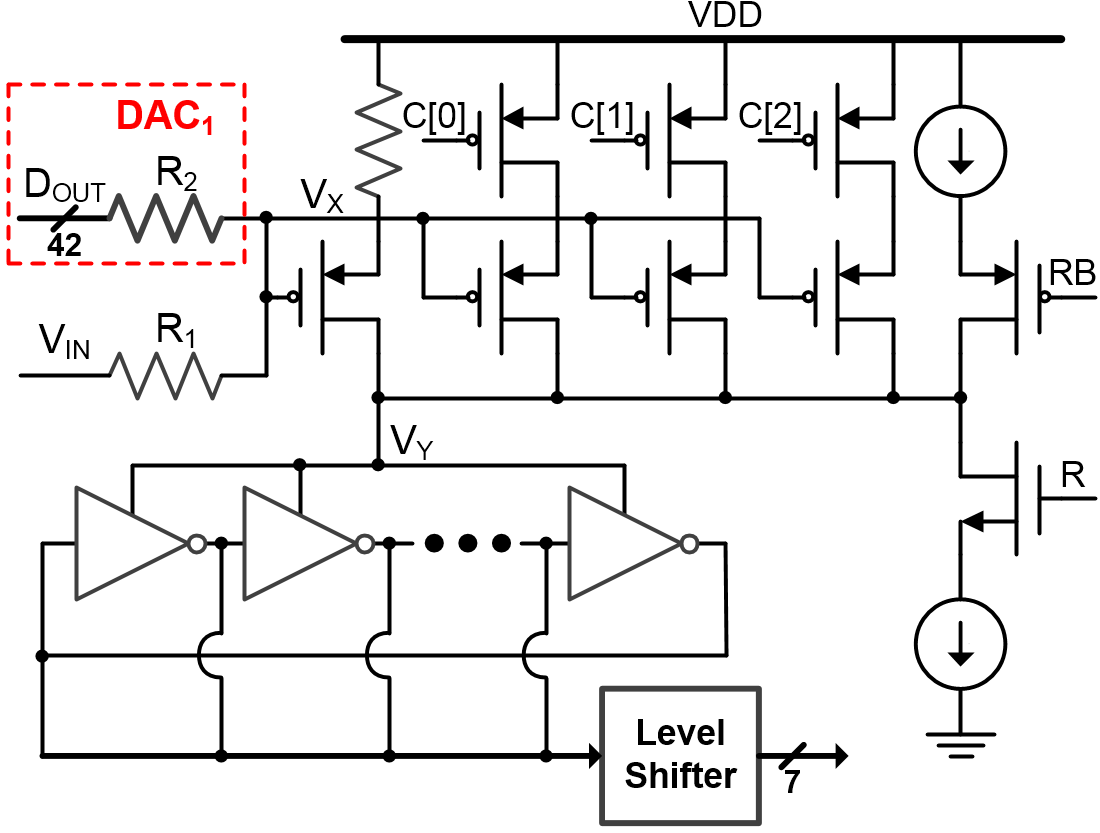##### (1)
$\frac{V_{I N}}{R_1}+\frac{\sum D_{O U T}}{R_2}=V_X\left(\frac{1}{R_1}+\frac{42}{R_2}\right)$

V$_{\mathrm{IN}}$ is the input voltage, and D$_{\mathrm{OUT}}$ is the digital thermometer output of the DSM. the R\-$_{2}$ is equal to the 32R$_{1}$ because the 10 bits, among the 42-bit output, are allocated for reset delay of the PFD$_{2}$, which will be mentioned in section \textsc{IV}-2. The VCO oscillates with a frequency proportional to the V$_{\mathrm{X}}$. The C[2:0] is added to control the gain of the VCO. Since the voltage swing range of the VCO’s multi-phase outputs is V$_{\mathrm{Y}}$, not VDD, level shifters are required to make the full-swing input of the PFD$_{1}$. The resonator outputs (R and RB) are connected for NTF optimization.

The schematic of the VCO in the 2$^{\mathrm{nd}}$-stage integrator is shown in Fig. 4. The inputs of the 2$^{\mathrm{nd}}$ stage, the PWM signal (PFD$_{1}$ output) and the digital thermometer code (DAC$_{2}$ and DAC$_{\mathrm{ELD}}$ output), are converted into the current by turning on the current sources directly. In order to generate the input PWM signal of the GRO-based quantizer only once in the sampling period, the V$_{\mathrm{CTRL}}$ controls the frequency of the differential VCOs. Like the 1$^{\mathrm{st}}$-stage VCO, the 2$^{\mathrm{nd}}$-stage VCO has a full-swing output by using the level shifter.

##### Fig. 4. The schematic of the VCO in the 2$^{\mathrm{nd}}$ stage integrator.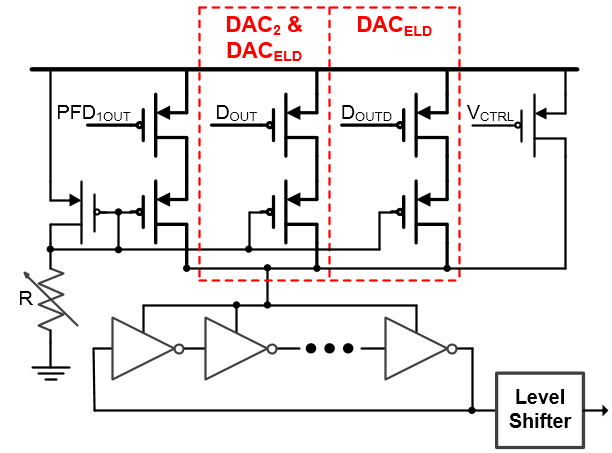### 2. GRO-based Quantizer

Thanks to the magnificent linearity and the intrinsic DWA, the GRO-TDC is utilized as the quantizer in various CTDSM [10,11]. Fig. 5 shows the structure of the GRO-TDC in the GRO-based quantizer. The GRO consists of 21 delay cells, which means that the GRO-TDC generates 21 digital thermometer outputs. The GRO oscillates when the input is high. After the GRO input becomes low, the GRO multi-phase outputs are sampled by the flip-flops. The sampled outputs are re-sampled so that the clock-to-Q delays of the 2$^{\mathrm{nd}}$ flip-flops are constant regardless of the GRO’s metastability.

##### Fig. 5. The GRO TDC structure.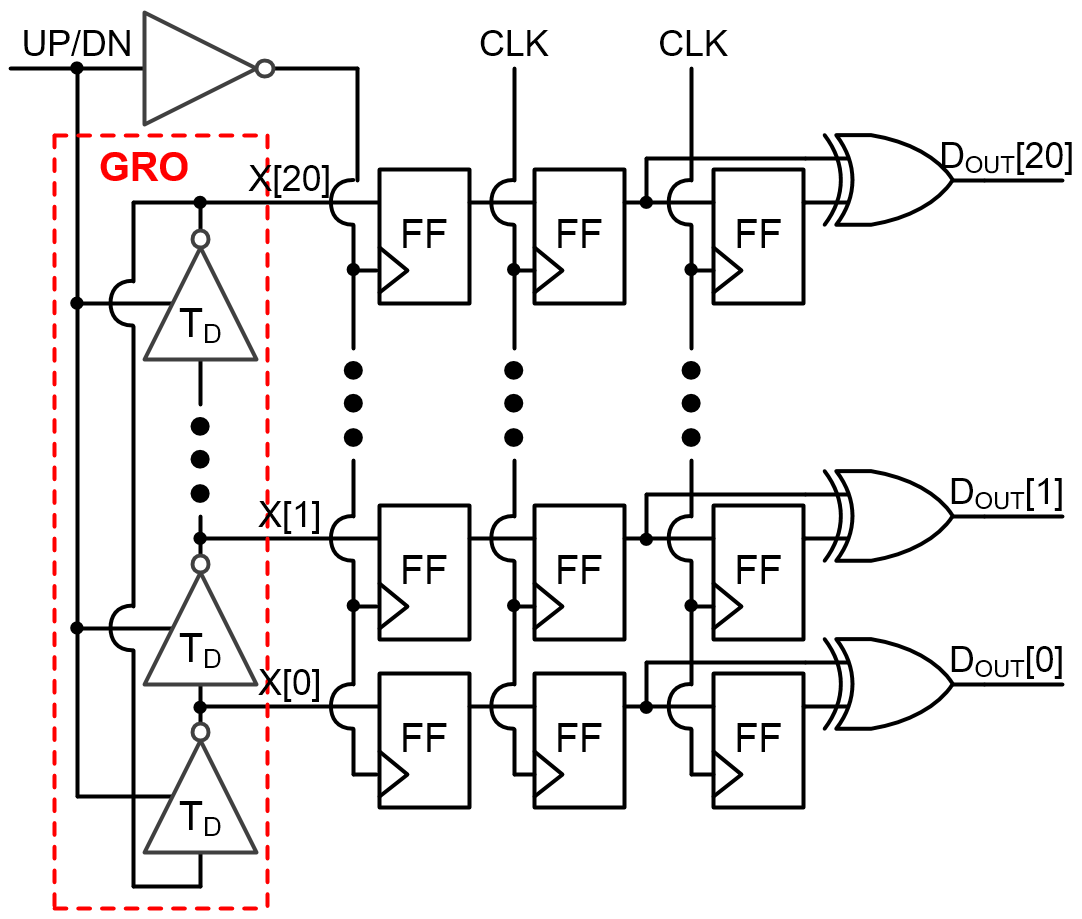The GRO-based quantizer consists of two GRO-TDCs whose input are the PFD$_{2}$ outputs, UP and DN. The pulse widths of these outputs are determined by not only the phase difference of the 2$^{\mathrm{nd}}$ differential VCOs but also the reset delay of the PFD$_{2}$. For example, if the rising edge of the VCO$_{\mathrm{P2}}$ is faster than that of the VCO$_{\mathrm{N2}}$, the pulse width of the UP is the sum of the rising edges difference and the reset delay, and that of the DN is equal to the reset delay. Therefore, the reset delay of the PFD2 is expressed as an offset in the output of the GRO-TDCs. In order for the GRO-TDC to be able to handle the full-range input, additional bits for reset delay are allocated. And all bits of the thermometer code including the additional bits should be fed back to the integrator through the DACs because all the bits can be 1 by the reset delay. The target resolution of the GRO-TDC is a 16-bit thermometer code which is equal to the 4-bit binary code, and 5-bit is allocated for each GRO-TDC to handle the reset delay.

### 3. The Frequency Controller

In order to generate one PWM input of the GRO-based quantizer during a sampling period, the center frequency of the 2$^{\mathrm{nd}}$-stage differential VCOs should be equal to the sampling frequency. Therefore, additional feedback is required to control the frequency of the 2$^{\mathrm{nd}}$-stage VCOs. The frequency controller, as shown in Fig. 6, has a structure similar to a phase-locked loop (PLL). When the rising edge of the VCO outputs (T$_{\mathrm{P}}$ and T$_{\mathrm{N}}$) is faster than the falling edge of the sampling clock (T$_{\mathrm{C}}$), the PFD makes the V$_{\mathrm{CTRL}}$ increase, and when the T$_{\mathrm{C}}$ is faster than the T$_{\mathrm{P}}$ and T$_{\mathrm{N}}$, the PFD makes the V$_{\mathrm{CTRL}}$ decrease. As a result, the V\-$_{\mathrm{CTRL}}$ is locked with a voltage that places the T$_{\mathrm{C}}$ in the center of the T$_{\mathrm{P}}$ and T$_{\mathrm{N}}$. That is, T$_{\mathrm{P}}$-T$_{\mathrm{C}}$ becomes equal to T$_{\mathrm{C}}$-T\-$_{\mathrm{N}}$.

##### Fig. 6. The architecture of the frequency controller and the waveform of its inputs and output.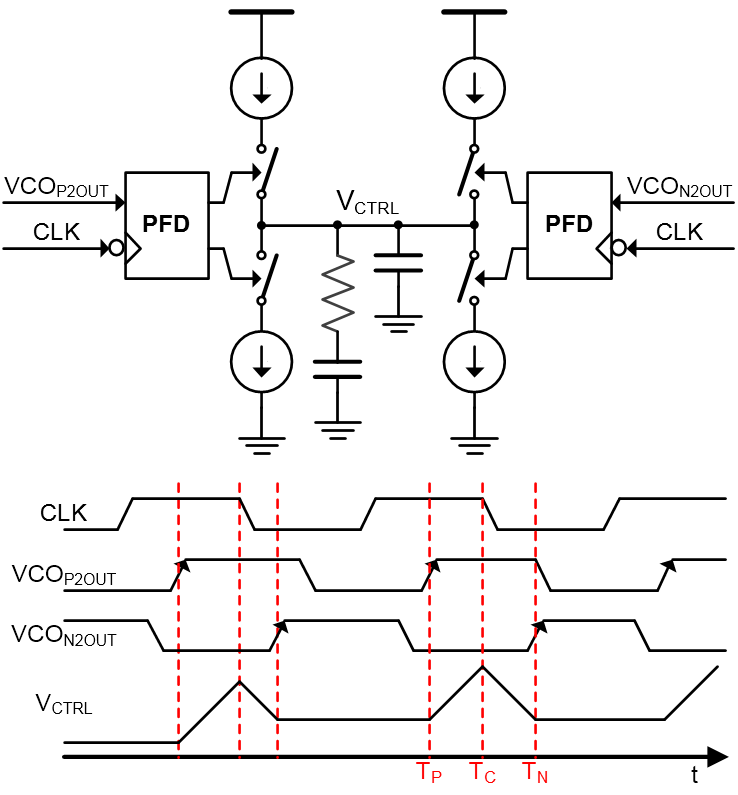## V. SIMULATION RESULTS

Fig. 7 shows the simulated power breakdown of the proposed CTDSM. The total power consumption is 3.84 mW, of which the power consumed by the VCOs and DACs accounts for 84 %. The frequency controller is necessary for adopting the GRO-based quantizer instead of the VCO-based quantizer. However, the power consumed by the frequency controller is not significant, less than 5%. The simulated FFT spectra are shown in Fig. 8. The SNDR of the proposed CTDSM is a 68.4 dB in the simulation including the analog noise such as thermal and flicker noise and 1% DAC mismatch at 1-MHz input frequency, 400-MHz sampling frequency, and 10-MHz bandwidth. A 1% DAC mismatch reduces the SNDR by 2.8 dB and introduces the harmonic distortion, but the effect of the harmonic distortion is not significant thanks to the intrinsic DWA. The NTF is optimized by the resonator, and then the noise level is flat from the low frequency to the 10-MHz and increases after that. Table 2 summarizes the specifications of the proposed CTDSM and the state-of-the-art CTDSMs.

##### Fig. 7. The simulated power breakdown of the proposed CTDSM.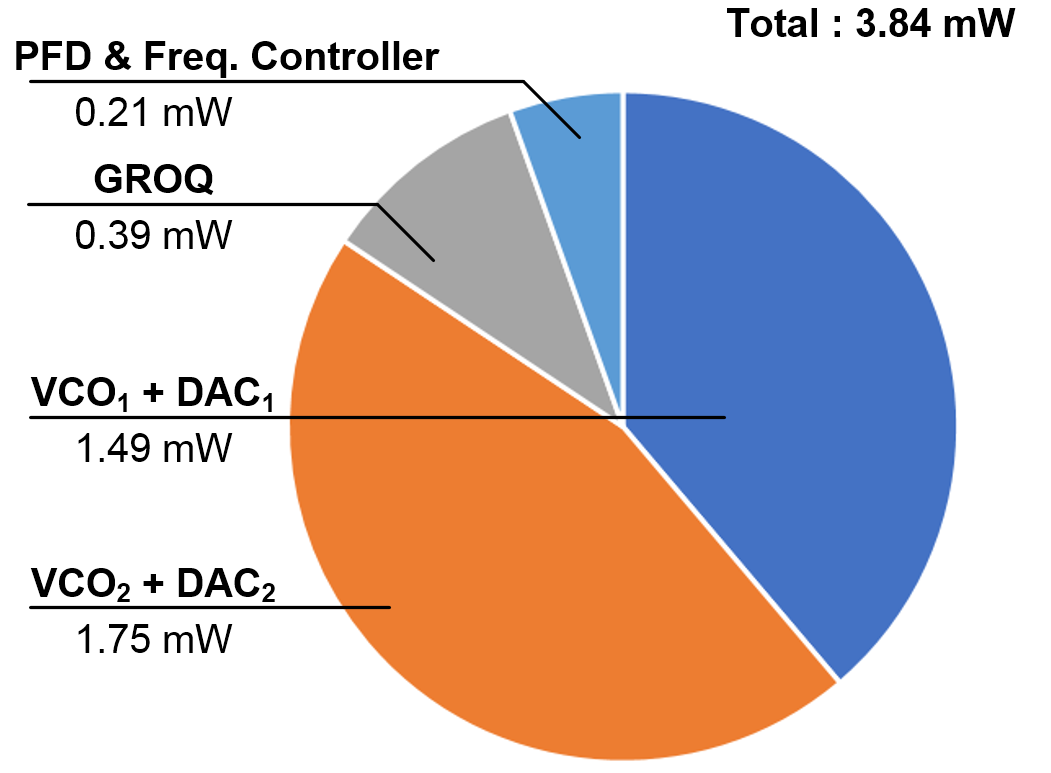##### Fig. 8. The Simulated output spectra.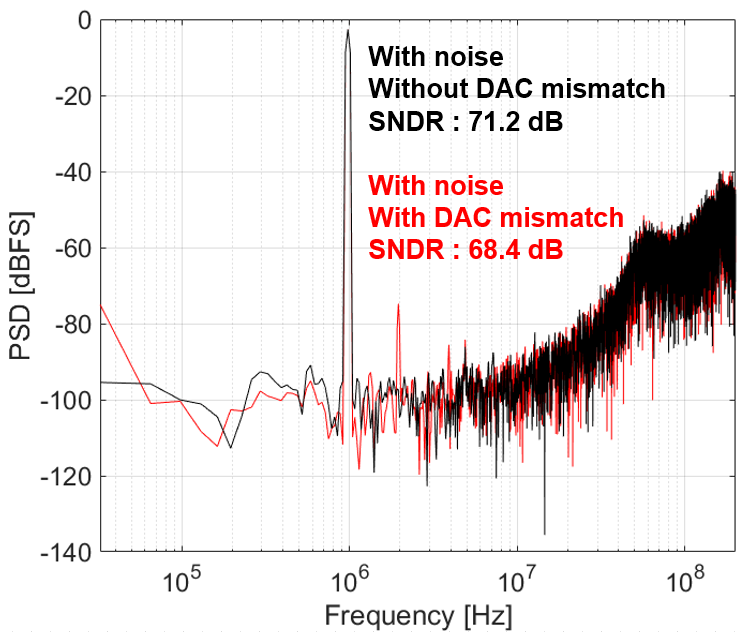##### Table 2. Simulated results of the proposed CTDSM
 Technology (nm) 28 Supply voltage (V) 0.9 Max. SNDR (dB) * 68.4 Sampling frequency (MHz) 400 Bandwidth (MHz) 10 Power consumption (mW) 3.84 FoMW (fJ/conv.) 89.3 FoMS (dB) 162.6
* The result includes the DAC mismatch and the analog noise.

## VI. CONCLUSIONS

This paper proposes the time-domain CTDSM using the VCO-based integrators and the GRO-based quantizer. The GRO-based quantizer achieves desirable characteristics: magnificent linearity, intrinsic DWA-based DEM, and 1$^{\mathrm{st}}$-order noise-shaping without the increase of the STF order. Although the input of the GRO-based quantizer is a PWM signal, the VCO-based integrator generates the input PWM signal of the quantizer without additional circuitry.

## ACKNOWLEDGMENTS

The research is sponsored in part by Samsung Research Funding & Incubation Center of Samsung Electronics under Project Number SRFC-IT2001-02, the National Research Foundation of Korea (NRF) grant funded by the Korea government (MSIT) (No. 2021R1C1C1003634), and the Industrial Strategic Technology Development Program (20013726) funded By the Ministry of Trade, Industry & Energy(MOTIE, Korea).

## References

1
Lee K., Yoon Y., Sun N., May, 2013, A 10MHz-BW, 5.6mW, 70dB SNDR ΔΣ ADC Using VCO-Based Integrators with Intrinsic DEM, 2013 IEEE International Symposium on Circuits and Systems (ISCAS), pp. 2006-20092
Straayer M. Z., Perrott M. H., Jun. 2007, A 10-bit 20MHz 38mW 950MHz CT ΔΣ ADC with a 5-bit noise-shaping VCO-based quantizer and DEM circuit in 0.13u cmos, 2007 IEEE Symposium on VLSI Circuits, pp. 246-2473
Park M., Perrott M. H., Dec. 2009, A 78 dB SNDR 87 mW 20 MHz Bandwidth Continuous-Time ΔΣ ADC With VCO-Based Integrator and Quantizer Implemented in 0.13 μm CMOS, IEEE Journal of Solid-State Circuits, Vol. 44, No. 12, pp. 3344-33584
Lee K., Yoon Y., Sun N., Dec. 2007, A Scaling-Friendly Low-Power Small-Area ΔΣ ADC With VCO-Based Integrator and Intrinsic Mismatch Shaping Capability, IEEE Journal on Emerging and Selected Topics in Circuits and Systems, Vol. 5, No. 4, pp. 561-5735
Pavan S., Nov. 2008, Excess Loop Delay Compensation in Continuous-Time Delta-Sigma Modulators, IEEE Transactions on Circuits and Systems-II, Vol. 55, No. 11, pp. 1119-11236
Kim T., C , Han , Maghari N., Aug. 2016, A 7.2 mW 75.3 dB SNDR 10 MHz BW CT Delta-Sigma Modulator Using Gm-C-Based Noise-Shaped Quantizer and Digital Integrator, IEEE Journal of Solid-State Circuits, Vol. 51, No. 8, pp. 1840-18507
Li S., Mukherjee A., Sun N., Jul. 2017, A 174.3-dB FoM VCO-Based CT ΔΣ Modulator With a Fully-Digital Phase Extended Quantizer and Tri-Level Resistor DAC in 130-nm CMOS, IEEE Journal of Solid-State Circuits, Vol. 52, No. 7, pp. 1940-19528
Zhong Y., et al. , Feb. 2020, A Second-Order Purely VCO-Based CT ΔΣ ADC Using a Modified DPLL Structure in 40-nm CMOS, IEEE Journal of Solid-State Circuits, Vol. 55, No. 2, pp. 356-3689
Maghami H., et al. , Mar. 2020, A Highly Linear OTA-Less 1-1 MASH VCO-Based ΔΣ ADC With an Efficient Phase Quantization Noise Extraction Technique, IEEE Journal of Solid-State Circuits, Vol. 55, No. 3, pp. 706-71810
Kim T., Han C., Maghari N., Dec. 2017, A 4th-Order Continuous-Time Delta-Sigma Modulator Using 6-bit Double Noise-Shaped Quantizer, IEEE Journal of Solid-State Circuits, Vol. 52, No. 12, pp. 3248-326111
Honarparvar M., de la Rosa J. M., Sawan M., Sep. 2020, A 10-MHz BW 77.3-dB SNDR 640-MS/s GRO-Based CT MASH ΔΣ Modulator, IEEE Transactions on Circuits and Systems-II, Vol. 67, No. 9, pp. 1519-1523Eunsang Lee (S’21) received his B.S. and M.S. degrees in Electrical Engineering from Hanyang University, Seoul, South Korea, in 2016, and 2018, respectively, and he is currently working towards the Ph. D. degree in electrical engineering. His research interests include ADC and ADC-based transceiver design.

Jaeduk Han (S’15–M’17) received his B.S. and M.S. degrees in Electrical Engineering from Seoul National University, Seoul, South Korea, in 2007, and 2009, respectively, and his Ph.D. degree in Electrical Engineering and Computer Sciences from University of California at Berkeley, CA, USA, in 2017. He has held various internship and full-time positions at TLI, Altera, Intel, Xilinx, and Apple, where he worked on digital, analog, and mixed-signal integrated circuit designs and design automations. He is currently an Assistant Professor of Electronic Engineering at Hanyang University, Seoul, South Korea. His research interests include high-speed analog and mixed-signal (AMS) circuit design and automation.